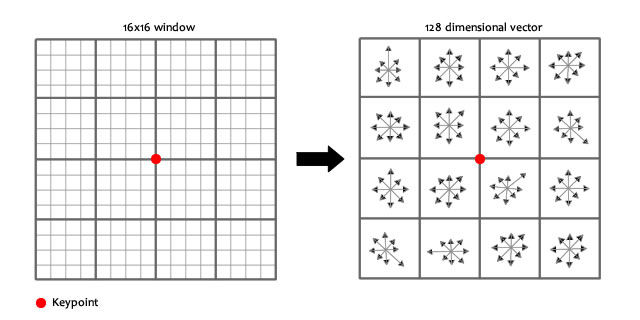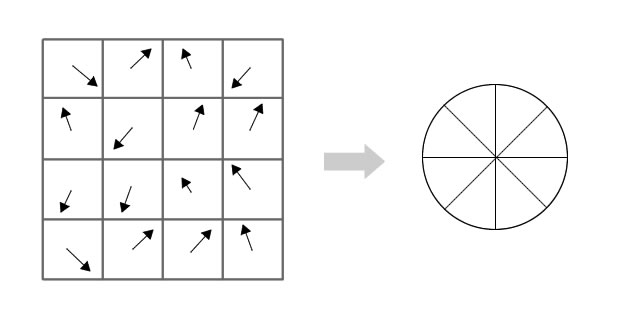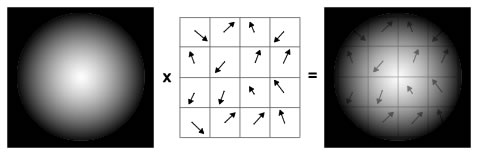## Generating a feature

Series: SIFT: Theory and Practice:

Now for the final step of SIFT. Till now, we had scale and rotation invariance. Now we create a fingerprint for each keypoint. This is to identify a keypoint. If an eye is a keypoint, then using this fingerprint, we'll be able to distinguish it from other keypoints, like ears, noses, fingers, etc.

## The idea

We want to generate a very unique fingerprint for the keypoint. It should be easy to calculate. We also want it to be relatively lenient when it is being compared against other keypoints. Things are never EXACTLY same when comparing two different images.

To do this, a 16x16 window around the keypoint. This 16x16 window is broken into sixteen 4x4 windows.Within each 4x4 window, gradient magnitudes and orientations are calculated. These orientations are put into an 8 bin histogram.Any gradient orientation in the range 0-44 degrees add to the first bin. 45-89 add to the next bin. And so on.And (as always) the amount added to the bin depends on the magnitude of the gradient.

Unlike the past, the amount added also depends on the distance from the keypoint. So gradients that are far away from the keypoint will add smaller values to the histogram.

This is done using a "gaussian weighting function". This function simply generates a gradient (it's like a 2D bell curve). You multiple it with the magnitude of orientations, and you get a weighted thingy. The farther away, the lesser the magnutide.Doing this for all 16 pixels, you would've "compiled" 16 totally random orientations into 8 predetermined bins. You do this for all sixteen 4x4 regions. So you end up with 4x4x8 = 128 numbers. Once you have all 128 numbers, you normalize them (just like you would normalize a vector in school, divide by root of sum of squares). These 128 numbers form the "feature vector". This keypoint is uniquely identified by this feature vector.

You might have seen that in the pictures above, the keypoint lies "in between". It does not lie exactly on a pixel. That's because it does not. The 16x16 window takes orientations and magnitudes of the image "in-between" pixels. So you need to interpolate the image to generate orientation and magnitude data "in between" pixels.

## Problems

This feature vector introduces a few complications. We need to get rid of them before finalizing the fingerprint.

1. Rotation dependence The feature vector uses gradient orientations. Clearly, if you rotate the image, everything changes. All gradient orientations also change. To achieve rotation independence, the keypoint's rotation is subtracted from each orientation. Thus each gradient orientation is relative to the keypoint's orientation.
2. Illumination dependence If we threshold numbers that are big, we can achieve achieve illumination independence. So, any number (of the 128) greater than 0.2 is changed to 0.2. This resultant feature vector is normalized again. And now you have an illumination independent feature vector!

## Summary

You take a 16x16 window of "in-between" pixels around the keypoint. You split that window into sixteen 4x4 windows. From each 4x4 window you generate a histogram of 8 bins. Each bin corresponding to 0-44 degrees, 45-89 degrees, etc. Gradient orientations from the 4x4 are put into these bins. This is done for all 4x4 blocks. Finally, you normalize the 128 values you get.

To solve a few problems, you subtract the keypoint's orientation and also threshold the value of each element of the feature vector to 0.2 (and normalize again).

## The End!

Once you have the features, you go play with them! I'll get to that in a later post(or posts :P). Read up on how the hough transform works. It will be used a lot.

Next, I'll try and explain an implementation of SIFT in OpenCV. Finally, some code! :D Though theory is :-~~

## More in the series

This tutorial is part of a series called SIFT: Theory and Practice:

Utkarsh Sinha created AI Shack in 2010 and has since been working on computer vision and related fields. He is currently at Microsoft working on computer vision.